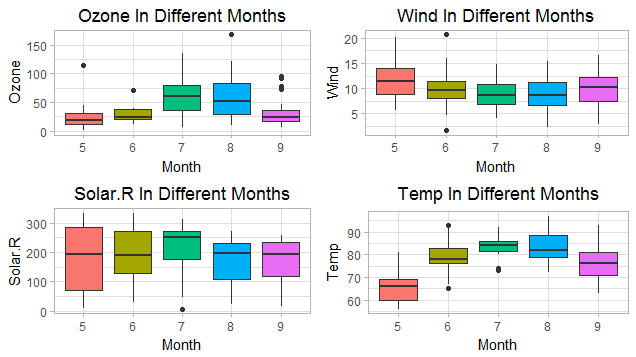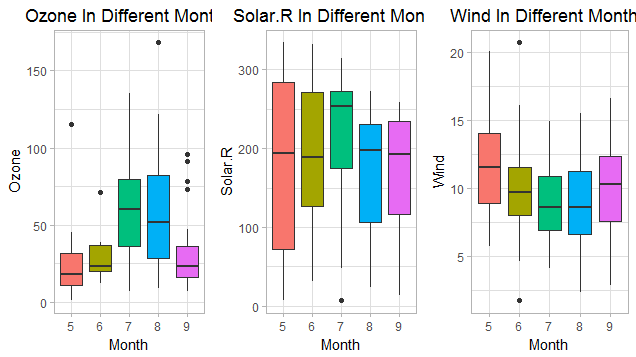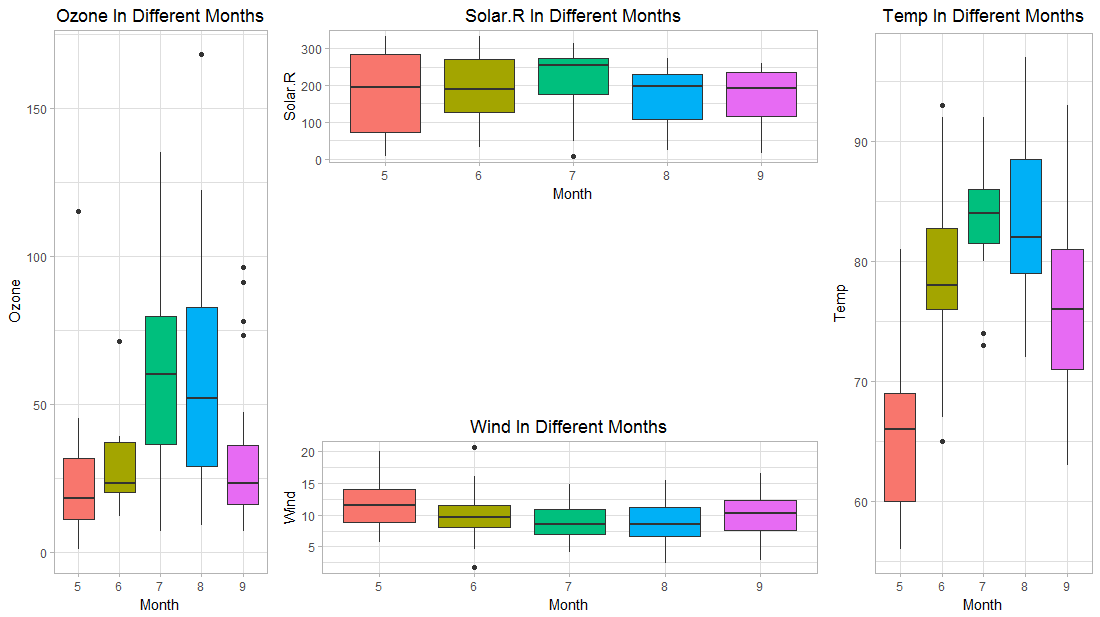R作图 ggplot2图片的布局排版

ggplot2是R语言中用来可视化的利器，其图层逻辑非常人性化，简单的说就是一句话一张图，后一张图叠加在前一张图上，所想表达的几乎都可以用一句代码在图形上展示出来。如果刚开始接触R作图的话，完全可以快速地从ggplot2入门而不用去管base作图，ggplot2的用法已经完全覆盖base作图，除了一项（我至今接触到的）——图片布局

data <- airquality
names(data)
# "Ozone"   "Solar.R" "Wind"    "Temp"    "Month"   "Day"

vars <- names(data)[1:4]
p <- lapply(vars, function(x){
ggplot(data, aes(x = Month, y = eval(parse(text = x)), fill = Month)) +
geom_boxplot(na.rm = T) +
labs(y = x, title = paste0(x, " In Different Months")) +
theme_light() +
theme(plot.title = element_text(hjust = 0.5)) +
guides(fill = "none")
})

p为列表，p[]代表第一张图

1. 用Rmisc包的multiplot函数

首先用最简单的方法做两张图

multiplot(plotlist = p[1:4], cols = 2)multiplot(plotlist = p[1:3], cols = 3)这里col表示每列的图片数目

我们还可以通过layout参数来设定图片的排布矩阵，如：

multiplot(plotlist = p[1:3], layout = matrix(c(1,2,3,3), nrow = 2))multiplot(plotlist = p[1:3], layout = matrix(c(1,2,3,3), nrow = 2, byrow = T))是不是很方便。。。。

2. 用grid包的viewport函数

grib包的viewport函数可以画上面的所有布局的图(PS.multiplot函数本来就是调用grib包的，所以当然都可以画出来)，但是有一种形式是multiplot函数无法比较方便的实现，但viewport函数可以（主要还是multiplot函数没封装完全，我看了下，重新改下函数，还是可以实现的），如下

先pushViewport设定一个空白画布，然后用pushViewport函数布局（主要用来设定后续viewport的可放置的位置）

library(grid)
grid.newpage()
pushViewport(viewport(layout = grid.layout(3,4)))

然后用print输出图片

print(p[], vp = viewport(layout.pos.row = 1:3, layout.pos.col = 1))
print(p[], vp = viewport(layout.pos.row = 1, layout.pos.col = 2:3))
print(p[], vp = viewport(layout.pos.row = 3, layout.pos.col = 2:3))
print(p[], vp = viewport(layout.pos.row = 1:3, layout.pos.col = 4))如果上述图片要用multiplot函数来实现，则需要这样写，PS.这矩阵写的好绕，还是viewport显得简洁了

multiplot(plotlist = p[1:4], layout = matrix(c(1,2,2,4,1,0,0,4,1,3,3,4), nrow = 3, byrow = T))